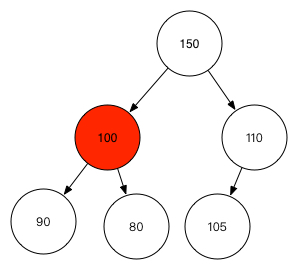jdk的优先队列使用PriorityQueue这个类，使用者可以自己定义优先级规则。

## 一个PriorityQueue例子

``````private static class Task {
String name;
int level;

public Task(String name, int level) {
this.name = name;
this.level = level;
}

public String getName() {
return name;
}

public void setName(String name) {
this.name = name;
}

public int getLevel() {
return level;
}

public void setLevel(int level) {
this.level = level;
}

@Override
public String toString() {
"name='" + name + '\'' +
", level=" + level +
'}';
}
}

public static void main(String[] args) {
@Override
return t2.getLevel() - t1.getLevel();
}
});
while(!queue.isEmpty()) {
System.out.println(queue.poll());
}
}
``````

``````Task{name='吃饭', level=100}
``````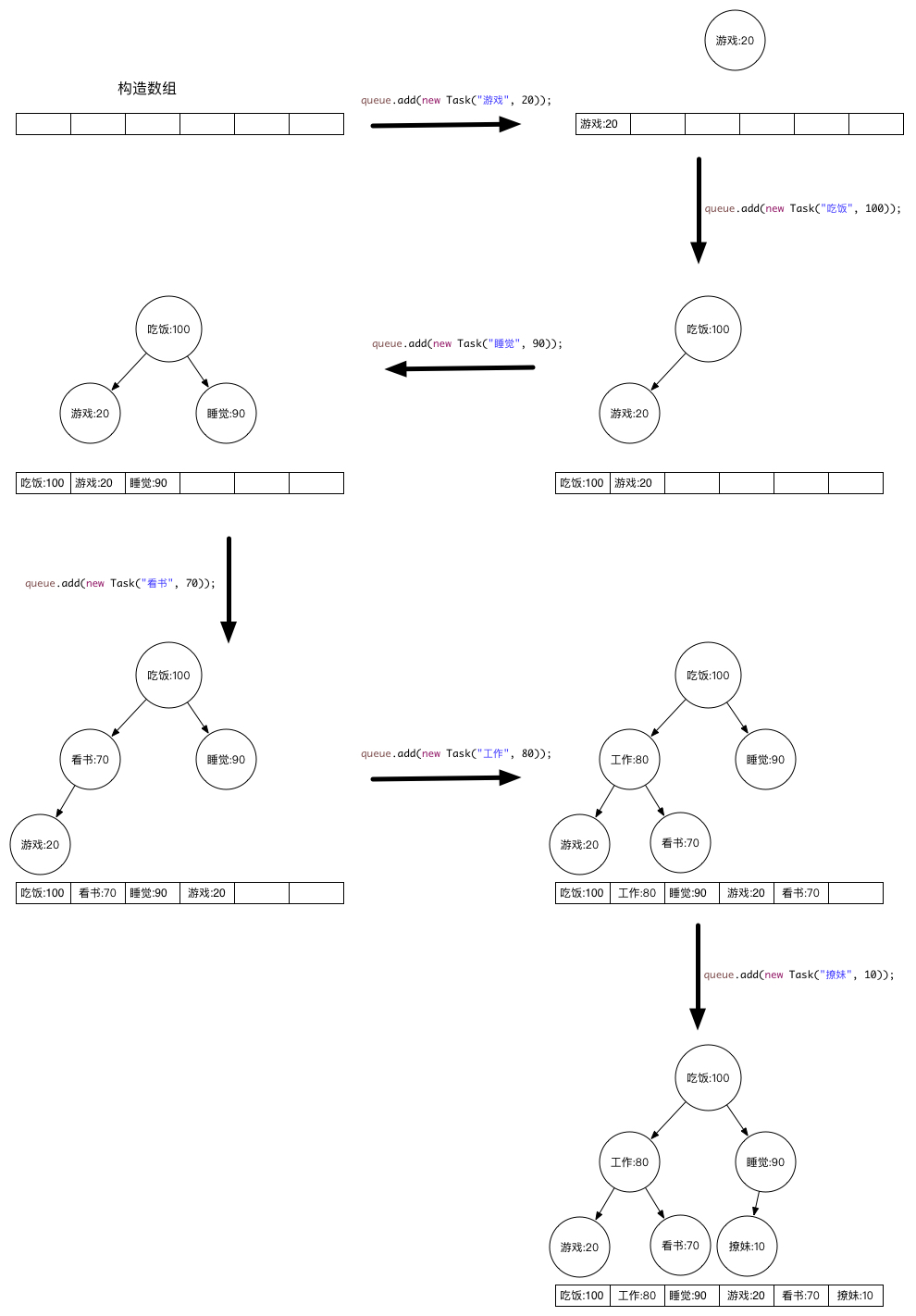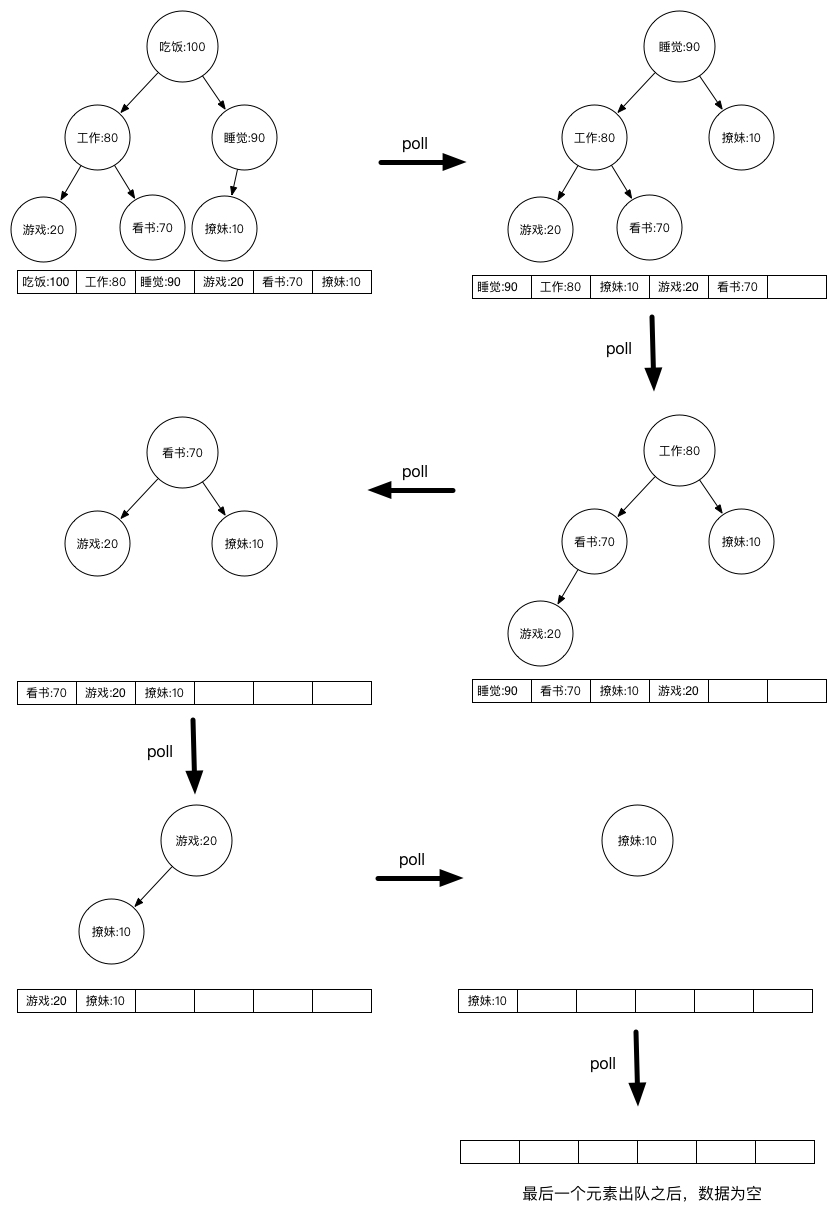## PriorityQueue原理分析

``````transient Object[] queue; // 堆
private int size = 0; // 元素个数
private final Comparator<? super E> comparator; // 比较器，如果是null，使用元素自身的比较器
``````

``````public boolean add(E e) {
}

public boolean offer(E e) {
if (e == null) // 元素为空的话，抛出NullPointerException异常
throw new NullPointerException();
modCount++;
int i = size;
if (i >= queue.length) // 如果当前用堆表示的数组已经满了，调用grow方法扩容
grow(i + 1); // 扩容
size = i + 1; // 元素个数+1
if (i == 0) // 堆还没有元素的情况
queue = e; // 直接给堆顶赋值元素
else // 堆中已有元素的情况
siftUp(i, e); // 重新调整堆，从下往上调整，因为新增元素是加到最后一个叶子节点
return true;
}

private void siftUp(int k, E x) {
if (comparator != null)  // 比较器存在的情况下
siftUpUsingComparator(k, x); // 使用比较器调整
else // 比较器不存在的情况下
siftUpComparable(k, x); // 使用元素自身的比较器调整
}

private void siftUpUsingComparator(int k, E x) {
while (k > 0) { // 一直循环直到父节点还存在
int parent = (k - 1) >>> 1; // 找到父节点索引
Object e = queue[parent]; // 赋值父节点元素
if (comparator.compare(x, (E) e) >= 0) // 新元素与父元素进行比较，如果满足比较器结果，直接跳出，否则进行调整
break;
queue[k] = e; // 进行调整，新位置的元素变成了父元素
k = parent; // 新位置索引变成父元素索引，进行递归操作
}
queue[k] = x; // 新添加的元素添加到堆中
}
``````

siftUp方法调用过程如下：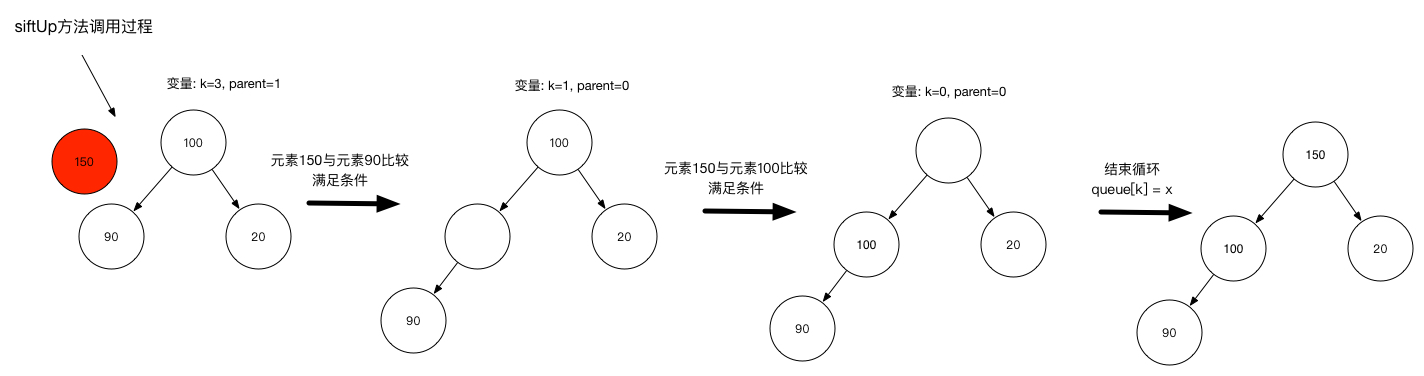poll，出队方法：

``````public E poll() {
if (size == 0)
return null;
int s = --size; // 元素个数-1
modCount++;
E result = (E) queue; // 得到堆顶元素
E x = (E) queue[s]; // 最后一个叶子节点
queue[s] = null; // 最后1个叶子节点置空
if (s != 0)
siftDown(0, x); // 从上往下调整，因为删除元素是删除堆顶的元素
return result;
}

private void siftDown(int k, E x) {
if (comparator != null) // 比较器存在的情况下
siftDownUsingComparator(k, x); // 使用比较器调整
else // 比较器不存在的情况下
siftDownComparable(k, x); // 使用元素自身的比较器调整
}

private void siftDownUsingComparator(int k, E x) {
int half = size >>> 1; // 只需循环节点个数的一般即可
while (k < half) {
int child = (k << 1) + 1; // 得到父节点的左子节点索引
Object c = queue[child]; // 得到左子元素
int right = child + 1; // 得到父节点的右子节点索引
if (right < size &&
comparator.compare((E) c, (E) queue[right]) > 0) // 左子节点跟右子节点比较，取更大的值
c = queue[child = right];
if (comparator.compare(x, (E) c) <= 0)  // 然后这个更大的值跟最后一个叶子节点比较

break;
queue[k] = c; // 新位置使用更大的值
k = child; // 新位置索引变成子元素索引，进行递归操作
}
queue[k] = x; // 最后一个叶子节点添加到合适的位置
}
``````

siftDown方法调用过程如下：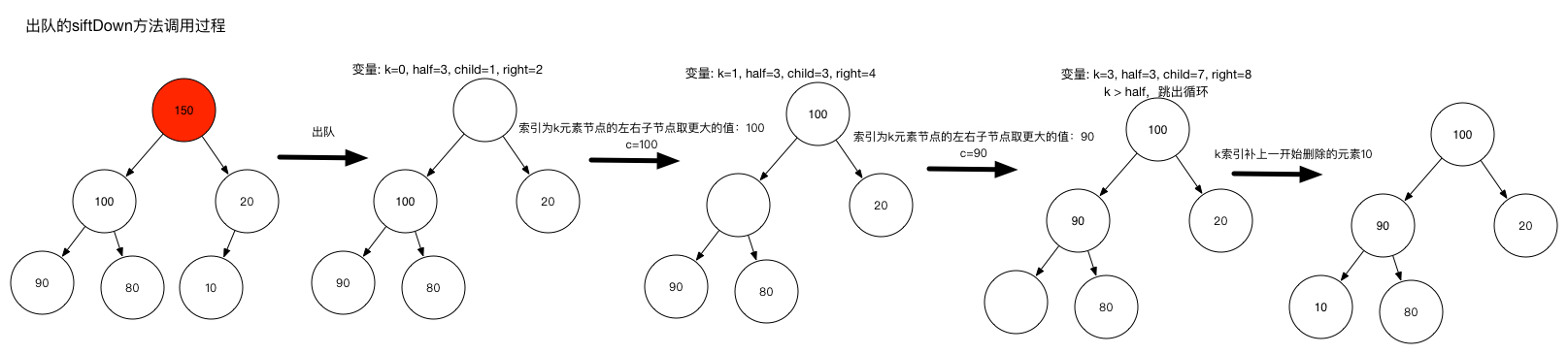grow扩容方法：

``````private void grow(int minCapacity) {
int oldCapacity = queue.length;
// 新容量
// 如果老容量小于64 新容量 = 老容量 + 老容量 + 2
// 如果老容量大于等于64 老容量 = 老容量 + 老容量/2
int newCapacity = oldCapacity + ((oldCapacity < 64) ?
(oldCapacity + 2) :
(oldCapacity >> 1));
// 溢出处理
if (newCapacity - MAX_ARRAY_SIZE > 0)
newCapacity = hugeCapacity(minCapacity);
// 使用新容量
queue = Arrays.copyOf(queue, newCapacity);
}
``````

remove，删除队列元素操作：

``````public boolean remove(Object o) {
int i = indexOf(o); // 找到数据对应的索引
if (i == -1) // 不存在的话返回false
return false;
else { // 存在的话调用removeAt方法，返回true
removeAt(i);
return true;
}
}

private E removeAt(int i) {
modCount++;
int s = --size; // 元素个数-1
if (s == i) // 如果是删除最后一个叶子节点
queue[i] = null; // 直接置空，删除即可，堆还是保持特质，不需要调整
else { // 如果是删除的不是最后一个叶子节点
E moved = (E) queue[s]; // 获得最后1个叶子节点元素
queue[s] = null; // 最后1个叶子节点置空
siftDown(i, moved); // 从上往下调整
if (queue[i] == moved) { // 如果从上往下调整完毕之后发现元素位置没变，从下往上调整
siftUp(i, moved); // 从下往上调整
if (queue[i] != moved)
return moved;
}
}
return null;
}
``````